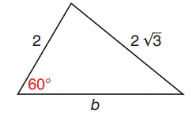Chapter 11.4, Problem 36EElementary Geometry For College St...

7th Edition
Alexander + 2 others
ISBN: 9781337614085

Solutions

Chapter
SectionElementary Geometry For College St...

7th Edition
Alexander + 2 others
ISBN: 9781337614085
Textbook Problem

For the triangle shown, use the Law of Cosines to determine b.To determine

To find:

The length b of the given triangle by using the law of cosines.

Explanation

Formula:

The Law of Cosines

a2=b2+c22bccosα

b2=a2+c22accosβ

c2=a2+b22abcosγ

Where α, β, and γ is the acute angle of the triangle, and a, b, and c is the sides of the triangle.

Calculation:

Given,

From the triangle,

Take a=2,c=23,andγ=60

By the law of cosines,

c2=a2+b22abcosγ

(23)2=(2)2+b22(2)bcos(60)

Since, cos60=12

4(3)=4+b2

Still sussing out bartleby?

Check out a sample textbook solution.

See a sample solution

The Solution to Your Study Problems

Bartleby provides explanations to thousands of textbook problems written by our experts, many with advanced degrees!

Get Started

Expand each expression in Exercises 122. x(3x+y)

Finite Mathematics and Applied Calculus (MindTap Course List)

Find the limit: limx2x38x2.

Calculus: An Applied Approach (MindTap Course List)

I=ER,E=120,andR=12.findI.

Elementary Technical Mathematics

The slope of the tangent line to y = x3 at x = 2 is: 18 12 6 0

Study Guide for Stewart's Single Variable Calculus: Early Transcendentals, 8th